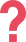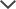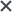## Cognitive Abilities Test Non-Verbal section

The CogAT Nonverbal Battery is used to assess a student’s reasoning abilities through the use of spatial and figural content. The test is useful for assessing the development of students who have trouble with reading, limited capability in English, or who have limited opportunities.

The subtests use geometric shapes and figures which have unlikely been seen by the students in their formal schooling. There is no reading required among the questions in the CogAT Nonverbal test. These three subtests are: Figure Matrices, Paper Folding, and Figure Classification. These three subtests combined make up the CogAT Nonverbal score.

## Number of Items by Level & Section

Nonverbal Battery Level 5/6 Level 7 Level 8 Level 9 Level 10-18
Figure Matrices 14 16 18 20 22
Paper Folding 10 12 14 16 16
Figure Classification 14 16 18 20 22

## Figure Matrices

Each question in this subsection portrays a 2 x 2 matrix. As in the Picture/Verbal Analogies in the CogAT Verbal Battery and the Number Analogies in the CogAT Quantitative Battery, the students must determine the relationship between the pictures in the two boxes in the top row and apply the same relationship in the bottom row. However, the Figure Matrices utilizes spatial forms. In all levels, students are presented with pictures in the matrices. This sub-test presents a relationship that is not always clear. Therefore, developing the skill to decipher the relationship is crucial to solve these types of questions. Below, you can see an example of two Figure Matrices questions from a Level 9 and Level 10 CogAT test:The correct answer is B. In the above analogy, as you move across the rows, the figures rotate clockwise 90 degrees (1/4 of a circle). Additionally, the colors of the figures are inversed. Parts of the figure which are white become black and parts of the figure which are black become white. The answer will exhibit the above analogous properties.

Tip: It is sometimes helpful to look at one part of the figure. For example, look at the single square in the top analogy. Observe how it rotates 1/4 of a circle in a clockwise direction and changes colors from black to white.Look closely at the top row from left to right:

In the left frame there is a circle with a vertical and a horizontal line inside. In the next frame (on the right), the circle shrinks and, in the center, overlaps the lines.

The same pattern should occur in the bottom row:

In the left frame, there is a diamond with two diagonal lines. In the next frame, the diamond should shrink and overlap the diagonal lines in the center of the frame.

Answer choice A is incorrect as the diamond is transparent and the lines can be seen.

Answer choice B is incorrect since the lines are no longer diagonal and the shape in the middle is a circle instead of a diamond.

Answer choice D is incorrect since the lines are not diagonal and there is no shape in the center.

Answer choices E is incorrect as it contains a black (not grey) diamond.

We are left with the answer choice C, which is the only correct answer.

Start Practicing for the CogAT Nonverbal Battery Now!

## Paper Folding

For Level 5/6 - Level 8, students must determine how a piece of paper will look once folded. In Levels 9 and up, the questions may include hole punching and require students to determine how the paper will appear when it is unfolded. This sub-test measures the spatial capabilities of a student. The ability to unfold the paper and foresee the resulting image can be indicative of a strong analytical mind. Below, you can see two examples of a Paper Folding questions from the CogAT test:The correct answer is C. First the paper was folded diagonally from the upper-left corner to the lower-right corner. Then it was folded again from the lower-left corner to the upper-right corner. Then three holes in different shapes were punched out. Therefore, when the paper is unfolded the holes will mirror each other across the diagonals of the page like this:To solve this question look for the answer choices in which the holes do not mirror each other in the right way. Answer choices B and D can be eliminated in this way. Next, focus on the area of each remaining answer choice that appears in the rightmost frame of the question series (in this case the triangular area on the right side) and eliminate all choices that are different. Answer choice A can be eliminated as the circular hole is under the pentagon hole but should be above it. E can also be eliminated as the pentagon has been rotated. We are left with C as the only correct answer.The square paper was first folded in half diagonally to create a triangle, and then folded again from each corner towards the middle of the triangle.

Then, four holes were cut out of the folded paper.

All four holes go through four layers of paper.

Therefore, the answer should have sixteen holes (4 holes x 4 layers).

The holes will flip over the fold each time the paper is unfolded – first upwards and to the left, and then diagonally.

Answer choices 1, 2, and 3 have less than 16 holes, so they can be eliminated.

Answer choice 4 does not mirror correctly the holes in the folded paper above.

We are left with the 5th answer choice, which is the correct answer.

Start Practicing for the CogAT Now!

## Figure Classification

Like the Picture/Verbal Classification in the CogAT Verbal Battery, students are presented with three figures and the student is to select the answer choice that is supposed to be the fourth figure of the set by determining how the figures and shapes are similar. The idea is to develop the skill of recognizing patterns and applying them to make a smart choice. Below, you can see an example of two Figure Classification questions from the CogAT test:The correct answer is A. In this question the three figures are all circles. The only answer choice that is a circle is A. The rest of the answers are all shapes that are different than the shapes in the question. Therefore, A is the correct answer.Each of the
three figures in this question includes:

• Two curly brackets,
• A circle,
• A triangle,
• A hexagon, and –
• A pentagon

This eliminates answer choice C, as it contains only one curly bracket.

Answer choice D can also be eliminated since it contains square brackets and not curly ones.

Answer choice B can be eliminated because it contains two triangles and two circles.

Answer choice E can be eliminated because it contains two squares.

We are left with answer choice A, which is the correct answer.

Access More CogAT Questions Like These!

Need HelpNeed Help